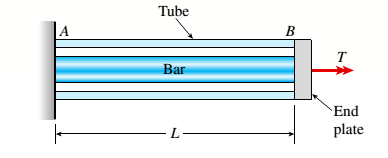# A solid steel bar of diameter d 1 = 25.0 mm is enclosed by a steel tube of outer diameter d 3 = 37.5 mm and inner diameter d 2 = 30.0 mm (see figure). Both bar and tube arc held rigidly by a support at end A and joined securely to a rigid plate at end B. The composite bar, which has a length L = 550 mm, is twisted by a torque T = 400 N ·m acting on the end plate. Determine the maximum shear stresses T 1 and T 2 in the bar and tube, respectively. Determine the angle of rotation 0(in degrees) of the end plate, assuming that the shear modulus of the steel is G = 80 GPa. Determine the torsional stiffness k T of the composite bar.### Mechanics of Materials (MindTap Co...

9th Edition
Barry J. Goodno + 1 other
Publisher: Cengage Learning
ISBN: 9781337093347### Mechanics of Materials (MindTap Co...

9th Edition
Barry J. Goodno + 1 other
Publisher: Cengage Learning
ISBN: 9781337093347
Chapter 3, Problem 3.8.12P
Textbook Problem
591 views

## A solid steel bar of diameter d1= 25.0 mm is enclosed by a steel tube of outer diameter d3= 37.5 mm and inner diameter d2= 30.0 mm (see figure). Both bar and tube arc held rigidly by a support at end A and joined securely to a rigid plate at end B. The composite bar, which has a length L = 550 mm, is twisted by a torque T = 400 N ·m acting on the end plate. Determine the maximum shear stresses T1and T2in the bar and tube, respectively. Determine the angle of rotation 0(in degrees) of the end plate, assuming that the shear modulus of the steel is G = 80 GPa.Determine the torsional stiffness kTof the composite bar.(a)

To determine

The maximum shear stresses in the bar.

The maximum shear stresses in the tube.

### Explanation of Solution

Given information:

The diameter of steel bar is 25mm , the outside diameter of steel tube is 37.5mm , the inside diameter of the steel tube is 30mm , the length of the composite bar is 550mm , twisted torque is 400Nm and the shear modulus of elasticity is 80×109N/m2 .

Write the expression for polar moment of inertia of bar.

Ip1=π32(d14) ...... (I)

Here, the polar moment of inertia of bar is IP , the diameter of bar is d1 .

Write the expression for polar moment of inertia of tube.

Ip2=π32(d34d24) ...... (II)

Here, the polar moment of inertia of bar is IP2 , the outside diameter of bar is d3 and the inside diameter of the tube is d2 .

Write the expression for torque in the bar.

Tb=T(Ip1Ip1+Ip2) ...... (III)

Here, the torque in the bar is Tb and the total twisted torque is T .

Write the expression for torque in the tube.

Tt=T(Ip2Ip1+Ip2) ...... (IV)

Here, the torque in the tube is Tt and the total twisted torque is T .

Write the expression for maximum shear stress in bar.

τ1=Tb(d12)Ip1...... (V)

Maximum shear stress in the bar is τ1 .

Write the expression for maximum shear stress in tube.

τ2=Tt(d32)Ip2...... (VI)

Maximum shear stress in the bar is τ1 .

Calculation:

Substitute 25mm for d1 in Equation (I).

Ip1=π32(25mm)4=π32(25mm( 1m 1000mm))4=π32(0.025m)4=3.834×108m4

Substitute 37.5mm for d3 and 30mm for d2in Equation (II).

Ip2=π32(( 37.5mm)4( 30mm)4)=π32(( 37.5mm( 1m1000mm ))4( 30mm( 1m1000mm ))4)=π32(1.97×106m48.1×107m4)=1.146×107m4

Substitute 1.146×107m4 for Ip2 , 3.834×108m4 for Ip1 and 400Nm in Equation (III).

Tb=(400Nm)(( 3.834× 10 8 m 4 )( 3

(b)

To determine

The angle of rotation of the end plate.

(c)

To determine

The torsional stiffness of the bar.

### Still sussing out bartleby?

Check out a sample textbook solution.

See a sample solution

#### The Solution to Your Study Problems

Bartleby provides explanations to thousands of textbook problems written by our experts, many with advanced degrees!

Get Started

Find more solutions based on key concepts
List and describe the different types of databases.

Database Systems: Design, Implementation, & Management

Describe factors that affect thermal comfort.

Engineering Fundamentals: An Introduction to Engineering (MindTap Course List)

What types of businesses use a dye-sublimation printer?

Enhanced Discovering Computers 2017 (Shelly Cashman Series) (MindTap Course List)

Name two types of respirators and describe how they work.

Welding: Principles and Applications (MindTap Course List)

If your motherboard supports ECC DDR3 memory, can you substitute non-ECC DDR3 memory?

A+ Guide to Hardware (Standalone Book) (MindTap Course List)